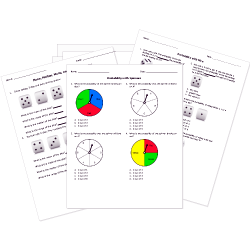Looking for Statistics worksheets?
Check out our pre-made Statistics worksheets!
 Tweet##### Browse Questions

You can create printable tests and worksheets from these Grade 7 Represent and Determine Probability questions! Select one or more questions using the checkboxes above each question. Then click the add selected questions to a test button before moving to another page.

Previous Next
Grade 7 Represent and Determine Probability CCSS: 7.SP.C.5
Probability can be expressed as a
1. fraction.
2. decimal.
3. percent.
4. all of these
Grade 7 Represent and Determine Probability CCSS: 7.SP.C.7, 7.SP.C.7a
Grade 7 Represent and Determine Probability CCSS: 7.SP.C.8, 7.SP.C.8a
A fair coin will be flipped 3 times. What is the probability that the coin will land on tails exactly once?
1. $1/8$
2. $1/3$
3. $3/8$
4. $5/8$
Grade 7 Represent and Determine Probability CCSS: 7.SP.C.5
Grade 7 Represent and Determine Probability CCSS: 7.SP.C.5
Grade 7 Represent and Determine Probability CCSS: 7.SP.C.8, 7.SP.C.8b
The set of all possible outcomes in a probability experiment.
1. Choices
2. Probability
3. Sample space
4. Outcome arrangements
Grade 7 Represent and Determine Probability CCSS: 7.SP.C.7, 7.SP.C.7a
Which scenario for the spinner has the following probability of occurring?
$P(?) = 2/3$1. The spinner lands on a number less than 2.
2. The spinner lands on a number less than 4.
3. The spinner lands on a number greater than 2.
4. The spinner lands on a number greater than 4.
Grade 7 Represent and Determine Probability CCSS: 7.SP.C.5
If you flip a coin, what is the probability that it will land heads up?
1. 1/2
2. .5
3. 50%
4. All of the above are equal and correct.
Previous Next
You need to have at least 5 reputation to vote a question down. Learn How To Earn Badges.